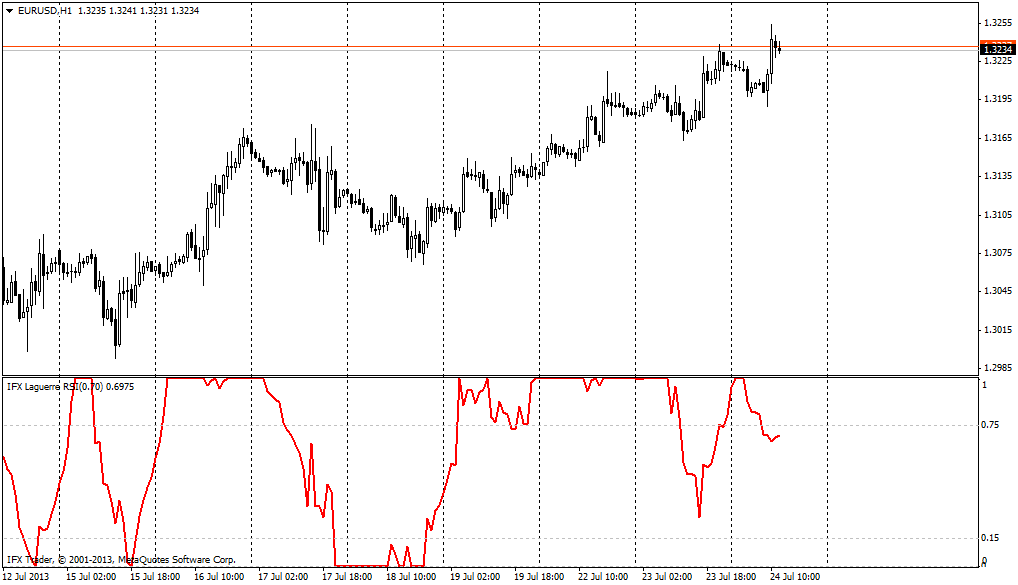You are about to be redirected to
www.instaforex.com
The services on this website better suit your location

#### Formula

L0:=((1-g)*C) + (g*PREV);

L1:=(-g*L0) + Ref(L0,-1) + (g*PREV);

L2:=(-g*L1) + Ref(L1,-1) + (g*PREV);

L3:=(-g*L2) + Ref(L2,-1) + (g*PREV);

cu:= If(L0 > L1, L0-L1,0) + If(L1 > L2, L1-L2,0) + If(L2 > L3, L2-L3,0);

cd:= If(L0 < L1, L1-L0,0) + If(L1 < L2, L2-L1,0) + If(L2 < L3, L3-L2,0).

As in case of many other oscillators, the main method of this indicator use is opening of buy orders after the level of indicator crosses above the level of 0.15 and opening of sell orders after the indicator crosses below the level of 0.75.

The major parameter of the RSI Laguerre indicator for MetaTrader is the factor gamma which equals 0.7 by default. It is important to note when the value which is less than this level (for instance, 0.5) is used, the indicator suggests rather aggressive trading with big number of trading signals, which nagatively influences the outcomes. High values of the indicator’s factor (more than 0.85) are unadvisable as overvaluation of the parameter may lead to delays in the work of the indicator.

The relevant distinguishing characteristic of the technical indicator RSI Laguerre is the situation when the indicator line takes minimal or maximal value moving strictly in horizontal way; it means powerful directed movement. Thus, if the indicator equals 1 and moves horizontally, strong trend movement, which is pointed up, prevails on the market. If the indicator equals zero and moves horizontally, then one should open sell deals in the developing downward movement.#### Parameters of InstaForex RSI Laguerre indicator

gamma = 0.7

You are now leaving www.instaforex.eu, a website operated by INSTANT TRADING EU LTD
Can't speak right now?